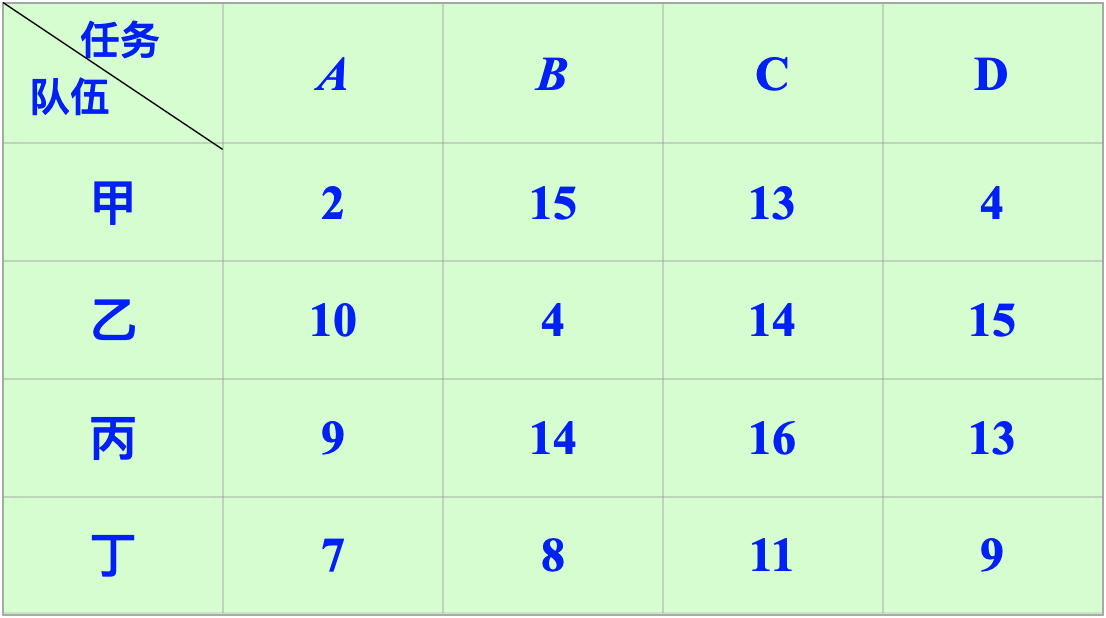# 一、前言

优化模型主要有线性规划、非线性规划、动态规划和整数规划。而指派问题是整数规划中一类重要的问题：
$n$项任务，由$n$个人来完成，每个人只能做一件，第$i$个人完成第$j$项任务要$c_{ij}$小时，如何合理安排时间才能使总用时最小？

# 二、 指派问题的数学模型

$x_{ij} = \begin{cases} 1, & \text{表示指派第i个人完成第j项工作} \\ 0, & \text{表示不指派第i个人完成第j项工作} \end{cases}$

$min \ z = \sum_{i=1}^n\sum_{j=1}^nc_{ij} x_{ij}$

$s.t = \begin{cases} \sum_{i=1}^n x_{ij}=1,\ \ \ \ \ i=1,2,···,n\\ \sum_{j=1}^n x_{ij}=1,\ \ \ \ \ j=1,2,···,n\\ x_{ij}=0或1,\ \ \ \ \ i,j = 1,2,···,n \end{cases}$

• 第一个式子表示完成全部n项工作所消耗的总资源数要最少；
• 第二个式子表示第i个人只完成一项工作；
• 第三个式子表示第j项工作只能由一个人完成；
• 第四个式子表示决策变量只能取0或者1。

# 三、 0 - 1规划求解(Matlab)c=[2,15,13,4,10,4,14,15,9,14,16,13,7,8,11,9]';
Aeq=[1,1,1,1,0,0,0,0,0,0,0,0,0,0,0,0;
0,0,0,0,1,1,1,1,0,0,0,0,0,0,0,0;
0,0,0,0,0,0,0,0,1,1,1,1,0,0,0,0;
0,0,0,0,0,0,0,0,0,0,0,0,1,1,1,1;
1,0,0,0,1,0,0,0,1,0,0,0,1,0,0,0;
0,1,0,0,0,1,0,0,0,1,0,0,0,1,0,0;
0,0,1,0,0,0,1,0,0,0,1,0,0,0,1,0;
0,0,0,1,0,0,0,1,0,0,0,1,0,0,0,1];
beq=[1;1;1;1;1;1;1;1];
lb=zeros(16,1);
ub=ones(16,1);
[x,fval] = linprog(c,[],[],Aeq,beq,lb,ub)
x=reshape(x,[4,4])'


c=[2,15,13,4;10,4,14,15;9,14,16,13;7,8,11,9];
c=c(:);a=zeros(8,16);intcon=1:16;
for i=1:4
a(i,(i-1)*4+1:4*i)=1;
a(4+i,i:4:16)=1;
end
b=ones(8,1);lb=zeros(16,1);ub=ones(16,1);
x=intlinprog(c,intcon,[],[],a,b,lb,ub)
x=reshape(x,[4,4])


posted @ 2021-06-09 23:02  很随便的wei  阅读(109)  评论(0编辑  收藏  举报